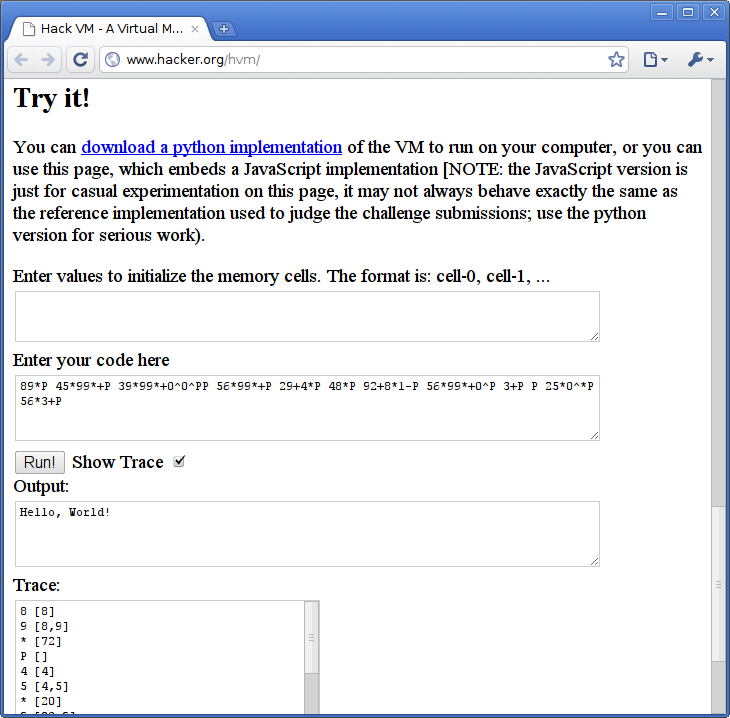# Miller's Hack VM (JavaScript)

Implementation of programming language Hack VM

One of the two original interpreters of Hack VM, written by its author Adam Miller. The implementation is written in JavaScript, and is stated to differ from Python one. It provides trace of execution — the state of memory stack after each command.Hack VM JavaScript interpreter

## Examples:

### Hello, World!:

Example for versions Miller's Hack VM (JavaScript), Miller's Hack VM (Python)

This program works in a rather evident way — ASCII-codes of characters of the message are calculated one-by-one and printed out. The only bit of hack used is processing of ‘l’ character — once its ASCII-code is calculated, it is tripled in the stack (using `0^` command), and printed twice immediately and once later.

``````89*P 45*99*+P 39*99*+0^0^PP 56*99*+P 29+4*P 48*P 92+8*1-P 56*99*+0^P 3+P P 25*0^*P 56*3+P
``````

### Fibonacci numbers:

Example for versions Miller's Hack VM (JavaScript), Miller's Hack VM (Python)

This example works much like the other Fibonacci numbers in esoteric languages: memory cell 0 stores the number of numbers left to calculate, cells 1 and 2 store ASCII-codes for comma and space, and cells 3 and 4 store two last calculated Fibonacci numbers. In a loop values of cells 3 and 4 are extracted, added together, the new value is printed, and storage cells are updated. After this, the number of numbers left is decremented, and if it’s 0, program counter (equivalent of instruction pointer in Brainfuck) is moved 6 cell forward, otherwise it jumps back to the start of the loop. Finally, three dots are printed.

``````27*0> 92+4*1> 84*2> 10^p3> 1<P 2<P 10^p4> 1<P 2<P 3< 4< + 0^p 4< 3> 4> 0< 1- 0> 0<  6? 67*c 58*6+0^0^PPP
``````

### Factorial:

Example for versions Miller's Hack VM (JavaScript), Miller's Hack VM (Python)

This example is pretty similar to Fibonacci numbers one, just with more characters to output. Note that data type is 32-bit integers, so 13! overflows: the output look like this:

0! = 1
1! = 1
2! = 2
3! = 6
4! = 24
5! = 120
6! = 720
7! = 5040
8! = 40320
9! = 362880
10! = 3628800
11! = 39916800
12! = 479001600
!ERROR: integer overflow

``````28*0> 56*3+1> 84*2> 78*5+3> 25*4> 05> 16> 5<p1<P2<P3<P2<P6<p4<P 5<1+5> 5<6<*6> 0<1-0> 0<6? 67*c
``````